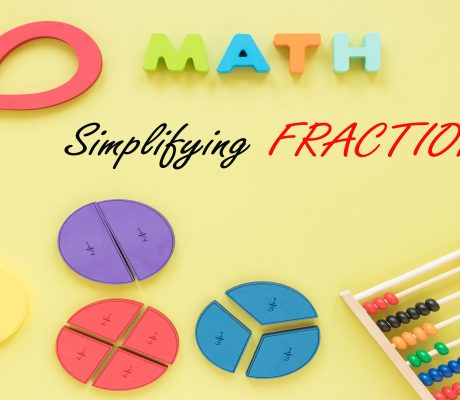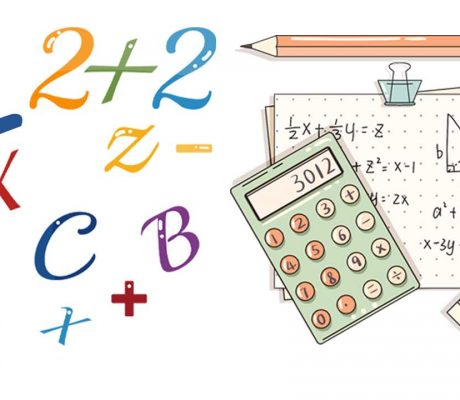# Tags: Equations### Learn ZOE Math Practice Questions with Answers

In this blog, there are 21 math practice questions that Learn ZOE teachers prepared for you. The questions are based on different grade levels and once the answer is revealed, there will be a detailed explanation of how we got the correct answer. Feel free to answer the math practice questions first before revealing the […]Adding and Subtracting fractions may seem a terrifying Math lesson for some, but the truth is it is not as complicated as some people might think. The trick is to be able to recognize and translate fractions into familiar objects; which is why pizza slices and chocolate bars are commonly used to represent fractions. This […]### Simplifying Fractions

What is a Fraction? A fraction represents a part of a whole. It describes equal parts of an object or a group like one-half, one-fourth, two-eighths, etc. For example, a slice of pizza is a fraction of the whole pizza. Some of these fractions can be simplified and we call this method simplifying fractions. The […]